Math Concepts

# Complete guide to Box and Whisker Plots

185 views

 1 Introduction 2 When to use a Box and Whisker Plot? 3 How to Make a Box and Whisker Plot? 4 Example 5 Interpreting a Boxplot 6 How to Read a Box Plot: Steps 7 Summary 8 Frequently Asked Questions 9 External References

23 November  2020

## Introduction

For some distributions/datasets, you will find that you need more information than the measures of central tendency (median, mean, and mode).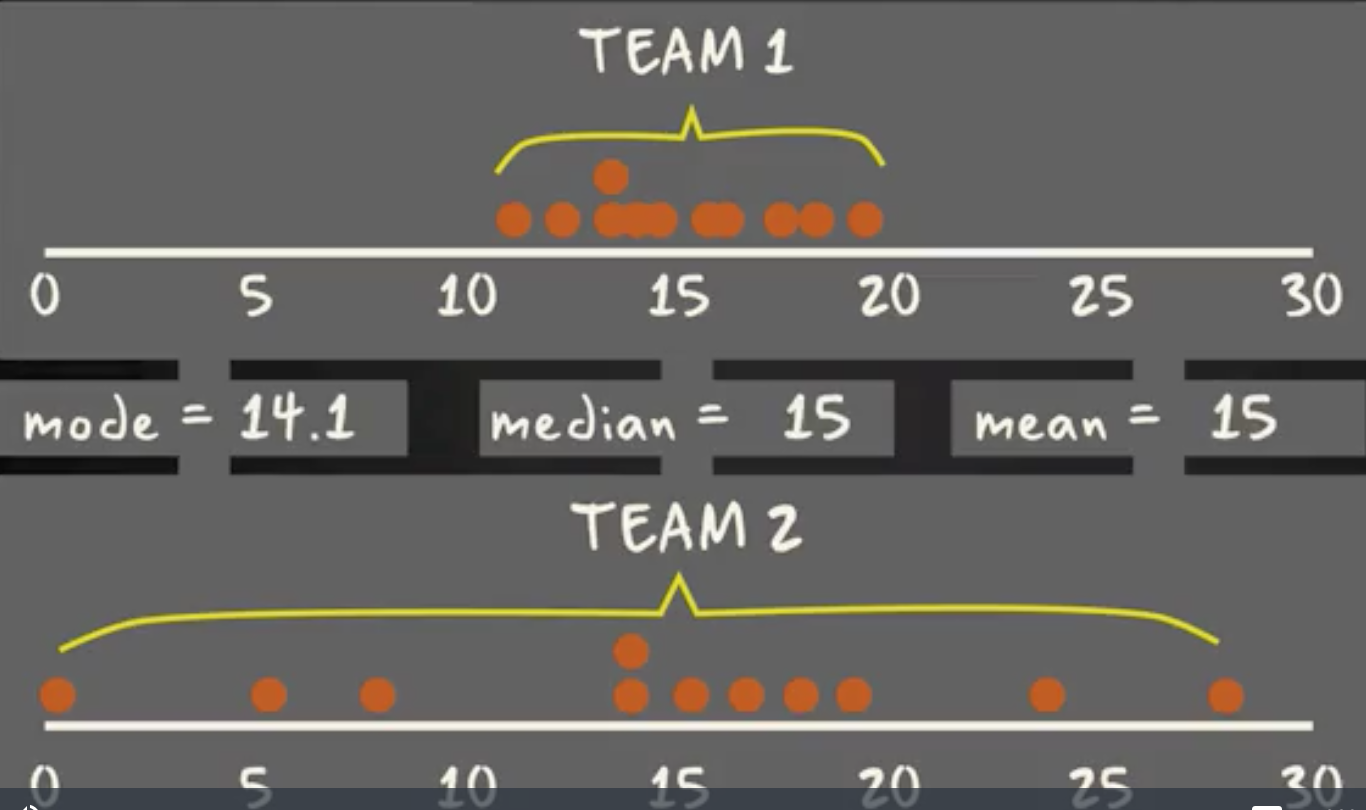You need to have information on the variability or dispersion of the data. A boxplot is a graph that gives you a good indication of how the values in the data are spread out. Although boxplots may seem primitive compared to a histogram or density plot, they have the advantage of taking up less space, which is useful when comparing distributions between many groups or datasets.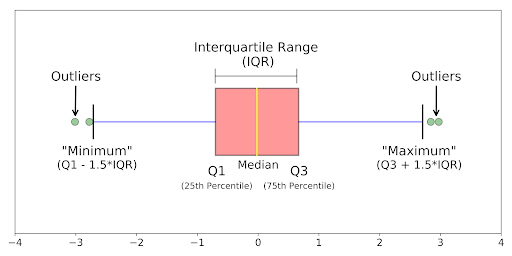Boxplots are a standardized way of displaying the distribution of data based on a five-number summary (“minimum”, first quartile (Q1), median, third quartile (Q3), and “maximum”).

Here are some additional points that talk about the Complete guide to Box and Whisker . To view them click on the Download button.

1. Median (Q2/50th Percentile): the middle value of the dataset.
2. First quartile (Q1/25th Percentile): the middle number between the smallest number (not the “minimum”) and the median of the dataset.
3. Third quartile (Q3/75th Percentile): the middle value between the median and the highest value (not the “maximum”) of the dataset.
4. Interquartile range (IQR): 25th to the 75th percentile.
5. Whiskers (shown in blue)
6. Outliers (shown as green circles)
7. “Maximum”: Q3 + 1.5*IQR
8. “Minimum”: Q1 -1.5*IQR

What defines an outlier, “minimum”, or“maximum” may not be clear yet. The next section will try to clear that up for you.

Data Handling Blogs
Representing Data Introduction to Graphs
Line and Bar Graphs Application Bar Plots and Line Graph Types
Histograms Frequency And Frequency Tables
Interquartile Range Graphs in Mathematics & Statistics
Mean Absolute Deviation Best Fit Line
Two-way Tables & Frequency Tables Venn Diagrams
Coronavirus Graphs Data Handling and its Types

## When to use a Box and Whisker Plot?

Use box and whisker plots when you have multiple data sets from independent sources related to each other in some way. Examples include:

• Test scores between schools or classrooms
• Data from before and after a process change
• Similar features on one part, such as camshaft lobes
• Data from duplicate machines manufacturing the same products

## How to Make a Box and Whisker Plot?

The procedure to develop a box and whisker plot comes from the five statistics below. You can also download the box and whisker plot template.

• Minimum value: The smallest value in the data set
• Second quartile: The value below which the lower 25% of the data are contained
• Median value: The middle number in a range of numbers
• Third quartile: The value above which the upper 25% of the data are contained
• Maximum value: The largest value in the data set

## Example

Suppose you wanted to compare three lathes’ performance responsible for the rough turning of a motor shaft. The design specification is 18.85 +/- 0.1 mm.
Diameter measurements from a sample of shafts taken from each roughing lathe are displayed in a box and whisker plot in the figure.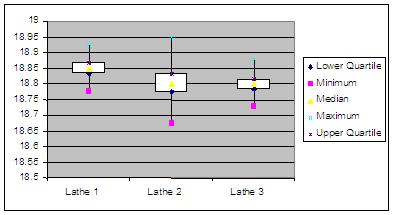• Lathe 1 appears to be making good parts and is centered in the tolerance.
• Lathe 2 appears to have excess variation and is making shafts below the minimum diameter.
• Lathe 3 performs with relatively less variation than Lathe 2; however, it is centered on the lower side of the specification and is making shafts below specification.

## Interpreting a Boxplot

Data science, Machine learning, and many other applicative mathematical fields are all about communicating results, so keep in mind you can always make your boxplots a bit prettier with a little bit of work.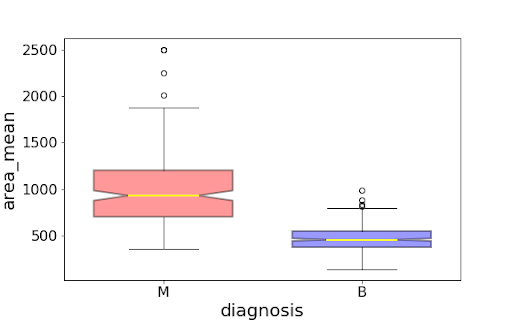Using the graph, we can compare the range and distribution of the area_mean for malignant and benign diagnosis. We observe that there is a greater variability for malignant tumor area_mean as well as larger outliers.

Also, since the notches in the boxplots do not overlap, you can conclude that with 95% confidence, that the actual medians do differ.

Here are a few other things to keep in mind about boxplots:

1. Keep in mind that you can always pull out the data from the boxplot if you want to know what the numerical values are for the different parts of a boxplot.
2. The median and the quartiles are calculated directly from the data. In other words, your boxplot may look different depending on the distribution of your data and the size of the sample, e.g., asymmetric and with more or fewer outliers.

A boxplot is a way to show a five-number summary in a chart. The main part of the chart (the “box”) shows where the middle portion of the data is: the interquartile range. At the ends of the box, you” find the first quartile (the 25%

mark) and the third quartile (the 75% mark). The far left of the chart (at the end of the left “whisker”) is the minimum (the smallest number in the set) and the far right is the maximum (the largest number in the set). Finally, the

median is represented by a vertical bar in the center of the box.

Box plots aren’t used that much in real life. However, they can be a useful tool for getting a quick summary of data.

## How to Read a Box Plot: Steps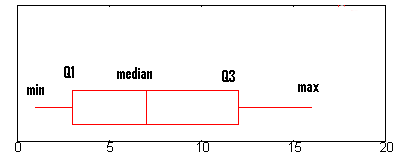Step 1: Find the minimum.

The minimum is the far left-hand side of the graph, at the tip of the left whisker. For this graph, the left whisker end is at approximately 0.75.

Step 2: Find Q1, the first quartile.

Q1 is represented by the far left-hand side of the box. In this case, about 2.5.

Step 3:  Find the median.

The median is represented by the vertical bar. In this boxplot, it can be found at about 6.5.

Step 4: Find Q3, the third quartile.

Q3 is the far right-hand edge of the box, at about 12 in this graph.

Step 5: Find the maximum.

The maximum is the end of the “whiskers”: in this graph, at approximately 16.

## Summary

This blog covered the topic of box and whisker plot. A box and whisker plot—also called a box plot—displays the five-number summary of a data set. The five-number summary is the minimum, first quartile, median, third quartile, and maximum.

In a box plot, we draw a box from the first quartile to the third quartile. A vertical line goes through the box at the median. The whiskers go from each quartile to the minimum or maximum.

Cuemath, student-friendly mathematics and coding platform, conducts regular Online Live Classes for academics and skill-development, and their Mental Math App, on both iOS and Android, is a one-stop solution for kids to develop multiple skills.

Check out the fee structure for all grades and book a trial class today!

## What is data?

Data are characteristics or information, usually numerical, that are collected through observation.

## How do you differentiate between data and information?

Data is the raw fact without any add on, but the information is derived from data.

Data Information
Raw facts of things Data with exact meaning
No contextual meaning Processed data and organized context
Just numbers and text

## What are the types of data?

There are two types of Data  :## What are the ways to represent data?

Tables, charts, and graphs are all ways of representing data, and they can be used for two general purposes. The first is to support the collection, organization, and analysis of data as part of a scientific study.

## What is a box and whisker plot?

A box and whisker plot—also called a box plot—displays the five-number summary of a data set. The five-number summary is the minimum, first quartile, median, third quartile, and maximum.

## External References

Related Articles
Award-winning math curriculum, FREE for a year